Game 27

Susan wanted to play the game. In the beginning, the first says a number from 1 to 8. Then the second player adds a number from 1 to 5 and tells the sum. Again, the Susan adds a number from 1-5 and say sum and etc. . . The winner must say the number 27. What first number did Susan have to say to definitely win?

Result

x =  3

Solution:Leave us a comment of example and its solution (i.e. if it is still somewhat unclear...):

Showing 1 comment:Bum
bum bum bumNext similar examples:

1. FactoryIn the factory workers work in three shifts. In the first inning operates half of all employees in the second inning and a third in the third inning 200 employees. How many employees work at the factory?
2. No. 215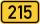From the number 215 we can create a four-digit number that among its numbers manually type any other digit. Thus, we created two four-digit numbers whose difference is 120. What two four-digit numbers that might be?
3. Solve 2Solve integer equation: a +b+c =30 a, b, c = can be odd natural number from this set (1,3,5,7,9,11,13,15)
4. Pages countingThere are pages numbered from 2 to 104 in the book. How many digits have to be printed to number the pages?
5. Summands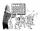Find two summands of the number 42, so that its product is minimized.
6. What is missing (1000)What number is to add to get 1000?
7. NumberWhat number is 20 % smaller than the number 198?
8. Identity123456789 = 100 Use only three plus or minus characters to correct previous identity/equation.
9. Dinning roomHow many different combinations can we choose if there are 3 soups, 5 kinds of main dish and 2 desserts in the dining room?
10. Bus 2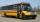On the 6-th stop 44 passengers take off from bus. Overall, the 6-th stop 13 passengers were added. How many passengers take on 6-th stop?Combine like terms 4c+c-7cPlace one pair of brackets in expression 20-16 + 10 - 4 - 2 so that the result will be 0.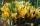The garden grow daffodils, crocuses and roses. 1400 daffodils, crocuses is 462 more and roses is 156 more than crocuses. How many are all the flowers in the garden?Steel pipe has a length 2.5 meters. About how many decimetres is 1/3 less than 4/8 of this steel pipe?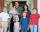The parents had 7 daughters. Each of them had one brother. How many members family had?Down syndrome is one of the serious diseases caused by a gene mutation. Down syndrome occurs in approximately every 550-born child. Express the incidence of Down's syndrome in newborns at per mille.In a class are 32 pupils. Of these are 8 boys. What percentage of girls are in the class?